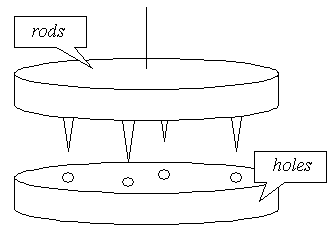# #255. 【Rujia Liu's Present 7】Xmas gift### 交互格式

1. Rotate k 表示将上半部顺时针旋转 $k$ 单位。
• 交互库不会返回任何信息。
2. Drop 表示尝试进行嵌合。
• 交互库会返回两部分的距离，即 $\max\{l_i-d_i\}$，$l_i$ 表示第 $i$ 根杆子的高度，$d_i$ 表示第 $i$ 个孔的深度。
• 如果交互库返回 $0$，交互库会立刻结束这组数据的评测。

### 样例

1 $~$
5 $~$
2 5 1 3 4 $~$
$~$ Rotate 4
$~$ Drop
3 $~$
$~$ Rotate 3
$~$ Drop
0 $~$

### 来源

Uva Online Judge，WC2003，题目作者刘汝佳。